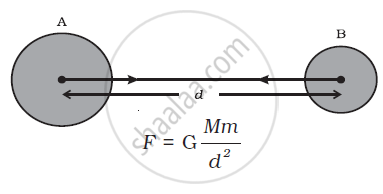# Newton’s Universal Law of Gravitation

• Newton’s universal law of gravitation
• Importance of the Universal Law of Gravitation
• Application of Newton’s law of gravitation
• Principle of superposition of gravitation

## Notes

IMPORTANCE OF THE UNIVERSAL LAW OF GRAVITATION

The universal law of gravitation successfully explained several phenomena which were believed to be unconnected:

(i) the force that binds us to the earth;

(ii) the motion of the moon around the earth;

(iii) the motion of planets around the Sun; and (iv) the tides due to the moon and the Sun.

## Notes

Universal Law of Gravitation

Every object in the universe attracts other object by a force of attraction, called gravitation, which is directly proportional to the product of masses of the objects and inversely proportional to the square of distance between them. This is called Law of Gravitation or Universal Law of Gravitation.

Let masses (M) and (m) of two objects are distance (d) apart. Let F be the attractional force between two masses.According to the universal law of gravitation, the force between two objects is directly proportional to the product of their masses. That is,

F∝M × m                             ....(1)

And the force between two objects is inversely proportional to the square of the distance between them, that is,

F ∝ 1/d                              ....(2)

Combining Eqs. (1) and (2), we get,

"F" ∝ ("M" xx "m") / "d"^2

or "F" = "G" ("M" xx "m")/"d"^2           ...(3)

where G is the constant of proportionality and is called the universal gravitation constant. By multiplying crosswise, Eq. (3) gives

F × d 2 = G M × m

or "G" = ("F""d"^2) / ("M" xx "m")      ...(4)

The SI unit of G can be obtained by substituting the units of force, distance and mass in Eq.(4) as N m2 kg–2. The value of G was found out by Henry Cavendish (1731 – 1810) by using a sensitive balance. The accepted value of G is 6.673 × 10–11 N m2 kg–2.

If you would like to contribute notes or other learning material, please submit them using the button below.

#### Video Tutorials

We have provided more than 1 series of video tutorials for some topics to help you get a better understanding of the topic.

Series 1

Series 2

### Shaalaa.com

Universal law of gravitation [00:17:59]
S
0%